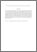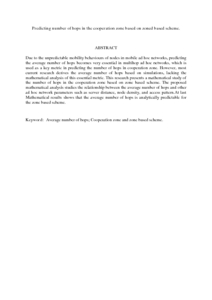Simple Search:

Predicting number of hops in the cooperation zone based on zoned based scheme.

Citation

Ibrahim, Saidu and Ahmad, Idawaty and Abdul Hamid, Nor Asila Waty and Yusuf, Mohammed waziri (2009) Predicting number of hops in the cooperation zone based on zoned based scheme. International Journal of Electrical and Computer Science, 9 (10). pp. 44-54. ISSN 2227-2739

Abstract / Synopsis

Due to the unpredictable mobility behaviours of nodes in mobile ad hoc networks, predicting the average number of hops becomes very essential in multihop ad hoc networks, which is used as a key metric in predicting the number of hops in cooperation zone. However, most current research derives the average number of hops based on simulations, lacking the mathematical analysis of this essential metric. This research presents a mathematical study of the number of hops in the cooperation zone based on zone based scheme. The proposed mathematical analysis studies the relationship between the average number of hops and other ad hoc network parameters such as server distance, node density, and access pattern.At last Mathematical results shows that the average number of hops is analytically predictable for the zone based scheme.Preview
PDF (Abstract)
Predicting number of hops in the cooperation zone based on zoned based scheme.pdfView Item